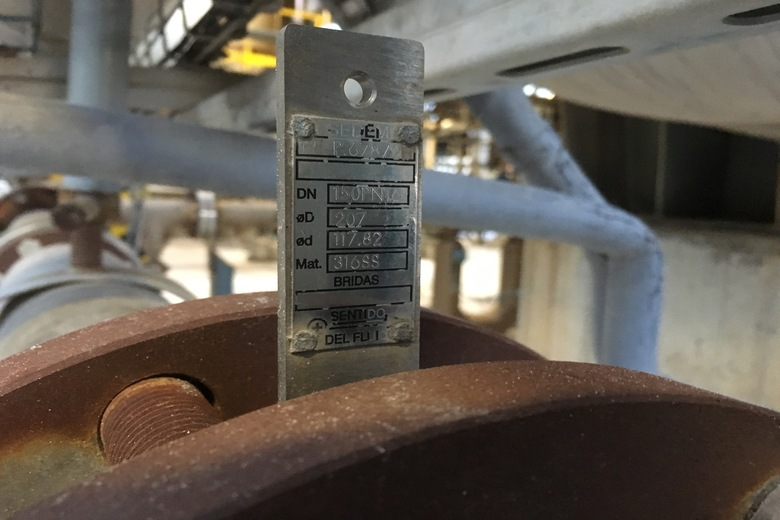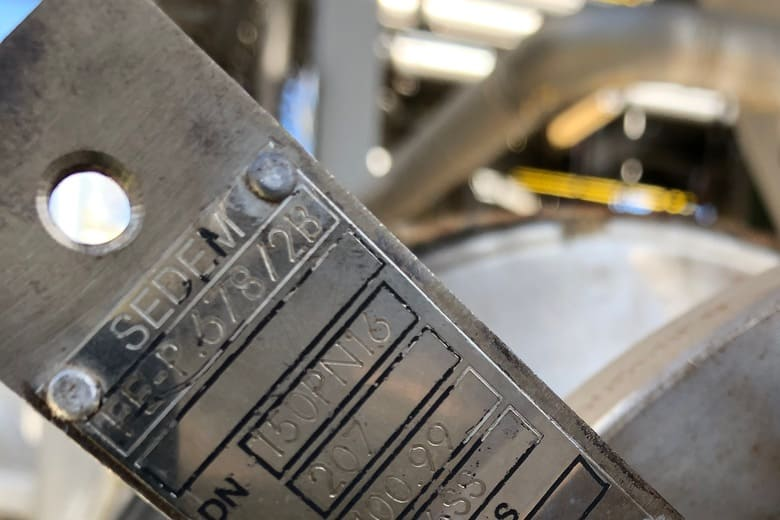# Restriction Orifice Calculators

## Our best restriction orifice calculators

In this category we will find useful tools for the instrumentation engineer, such as the measurement of the different variables that allow defining a restriction orifice. With these calculators we can determine the dimension of the orifice size, the pressure drop or the volumetric flow. We will gradually add more tools to this section. The results of all calculators are exportable in spreadsheet format. If you have any questions or require further clarification, do not hesitate to contact us.#### Restriction Orifice Calculator - Find Flow Rate

This calculator let you calculate the flow rate based produced by a restriction orifice plate. The results are exportable in spreadsheet format.#### Restriction Orifice Calculator - Find Orifice Size

This calculator let you calculate the restriction orifice size based on pressure drop and flow rate. The results are exportable in spreadsheet format.#### Restriction Orifice Calculator - Find Pressure Drop

This calculator let you calculate the restriction orifice pressure drop starting from orifice size and flow rate. The results are exportable in spreadsheet format.